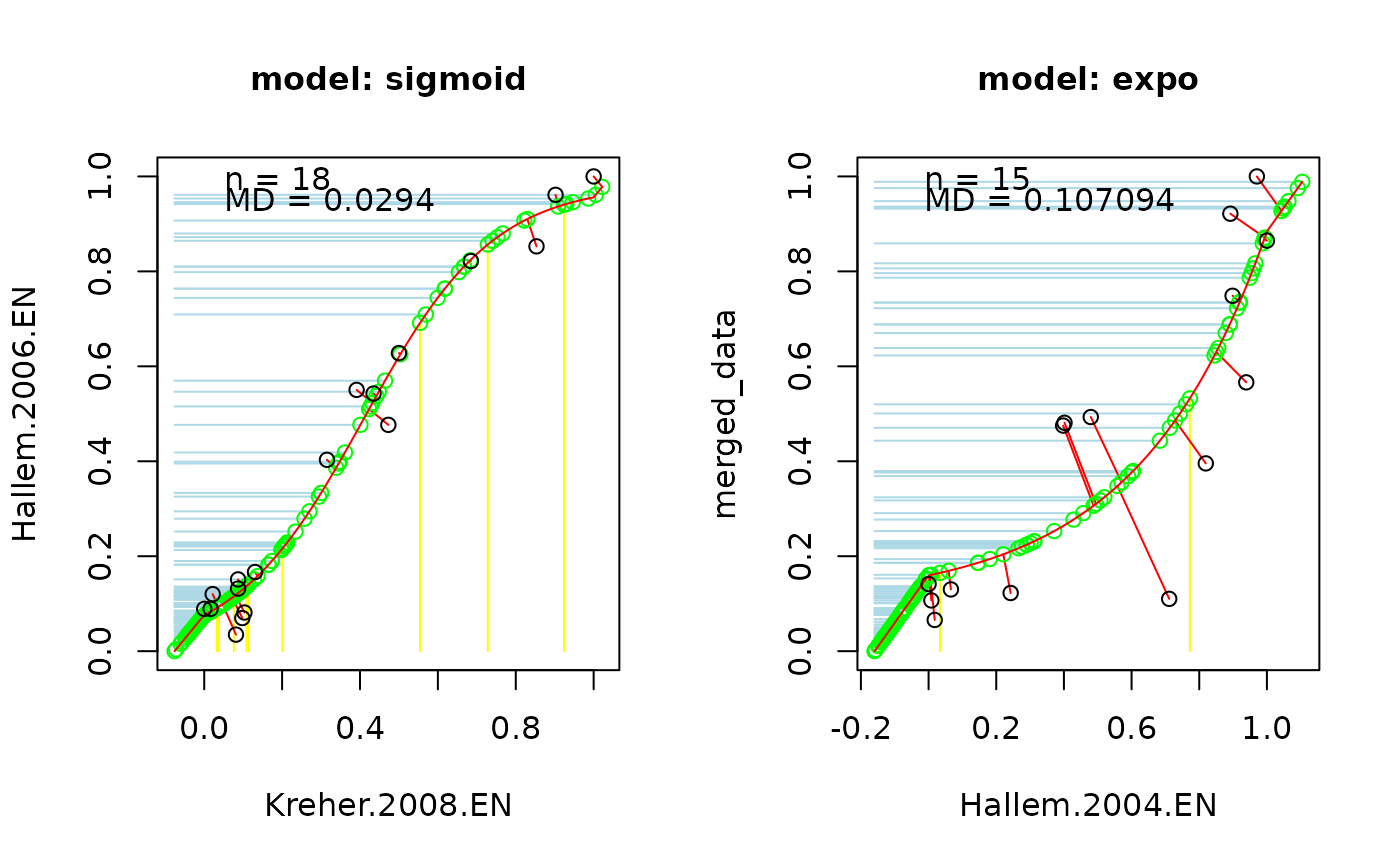Runs the DoOR algorithm, that merges all measurements for one receptor into a common response model.

## Usage

``````model_response(da, select.MDValue = door_default_values("select.MDValue"),
overlapValues = door_default_values("overlapValues"),
responseRange = door_default_values("door_response_range"),
weightGlobNorm = door_default_values("door_global_normalization_weights"),
glob.normalization = door_default_values("glob.normalization"),
plot = door_default_values("plot"))``````

## Arguments

da

data frame, a selected receptor containing measured responses from studies.

select.MDValue

numeric, threshold on the MD for rejecting a fit.

overlapValues

numeric, a criterion using to refuse a data set that has not enough overlap value.

responseRange

data frame, contains response ranges for all studies.

weightGlobNorm

data frame, a binary data matrix, 1 indicates given odor has been measured in given study, NA indicates NOT.

glob.normalization

logical, default is `TRUE`, performs a global normalization for the model response. Otherwise (`FALSE`) response values will be given in value from 0 to 1.

plot

logical, If `FALSE`, plotting is suppressed. Default is `FALSE`.

## Details

Merging a data is processed by following:

1. Normalize all response data in value [0,1].

2. Compute the correlation between studies and selected the best pair using `select_model`.

3. Merge the first pair using function `project_points`.

4. Add other datasets if the correlation between the growing model response and the new dataset is below the correlation threshold (select.MDValue). Datasets excluded based on this criterion will be appended in a separate list.

## Author

Shouwen Ma <shouwen.ma@uni-konstanz.de>

## Examples

``````# load data
library(DoOR.data)
data(Or35a)
data(door_global_normalization_weights)
data(door_response_range)

# merge all existing data sets for Or35a into a consensus model response
model_response_Or35a <- model_response(Or35a, plot = TRUE)
#> da: REMOVED Montague.2011.EN as overlap with all other studies was smaller than 5!``````